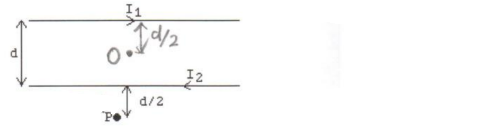# Problem: In the figure, the two long straight wires are separated by a distance of d = 0.40 m. The currents are I1 = 1.0 A to the right in the upper wire and I 2 = 8.0 A to the left in the lower wire. Find the magnitude and direction of the magnetic field at the center point O, and also point P, a distance d/2 = 0.20 m below the lower wire? (NOTE: μ0 = 4π x 10-7 T•m/A.)

###### FREE Expert Solution
81% (246 ratings)
###### Problem Details

In the figure, the two long straight wires are separated by a distance of d = 0.40 m. The currents are I1 = 1.0 A to the right in the upper wire and I 2 = 8.0 A to the left in the lower wire. Find the magnitude and direction of the magnetic field at the center point O, and also point P, a distance d/2 = 0.20 m below the lower wire? (NOTE: μ0 = 4π x 10-7 T•m/A.)Frequently Asked Questions

What scientific concept do you need to know in order to solve this problem?

Our tutors have indicated that to solve this problem you will need to apply the Magnetic Field Produced by Straight Currents concept. You can view video lessons to learn Magnetic Field Produced by Straight Currents. Or if you need more Magnetic Field Produced by Straight Currents practice, you can also practice Magnetic Field Produced by Straight Currents practice problems.

How long does this problem take to solve?

Our expert Physics tutor, Juan took 6 minutes and 8 seconds to solve this problem. You can follow their steps in the video explanation above.

What professor is this problem relevant for?

Based on our data, we think this problem is relevant for Professor Lumata's class at UTD.